Prev Up Next

The following puzzle was posed by Jeff and discussed by Lummox JR.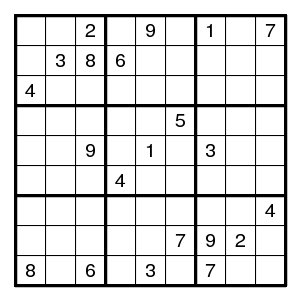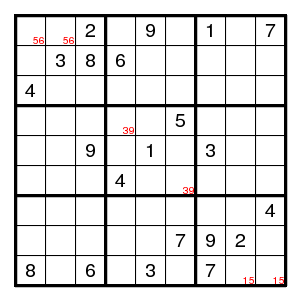Easy steps only give three double pairs, and then more is needed.

## Swordfish

The next step is a swordfish: the digit 4 in columns 3,5,7 can only be in rows 2,4,8, so does not occur elsewhere in these rows.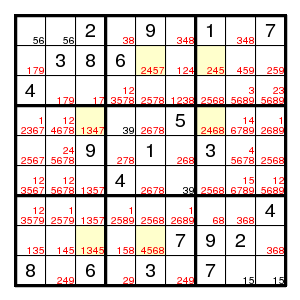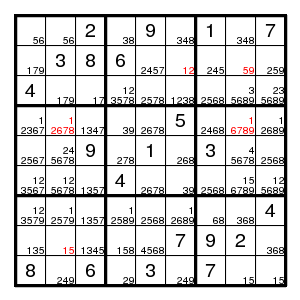## Colouring

Lummox JR proposes to do colouring for all digits. Since that requires too many colours to work with, designate the colours by letters, where upper and lower case denote what earlier were the darker and lighter shades: two possibilities of which precisely one must be correct.

In the grid below we repeat the possibilities just found, and write letters below some of the possibilities. (Which? Each time there is a bivalue, like the 56 in (1,1) and each time there is a pair, like the 4 in row 1 that must be in either (1,6) or (1,8), it is designated by a new upper/lower case letter, unless some element of the pair has a letter already. We find 17 colours. Each colour except c/C touches two cells only.)

`56aA`
`56Aa`
2
`38bB`
9
`348 c `
1
`348 C `
7
`179def`
3 8 6
`2457 C E`
`12Dd`
`245 c `
`59gG`
`259`
4
`179  F`
`17hH`
`123578    i `
`2578`
`1238`
`2568`
`35689`
`235689 j    `
`12367`
`12678`
`1347  c `
`39kK`
`2678`
5
`2468 C  `
`16789`
`12689`
`2567`
`245678 C    `
9
`278 I `
1
`268 l `
3
`45678c    `
`2568`
`123567`
`125678`
`1357`
4
`2678`
`39Kk`
`2568`
`156789`
`125689`
`123579     F`
`12579`
`1357`
`12589`
`2568`
`12689  L  `
`68mM`
`368j  `
4
`135`
`15nN`
`1345  C `
`158`
`4568c o `
7 9 2
`368JO `
8
`249 c `
6
`29pP`
3
`249 C `
7
`15qQ`
`15Qq`

Now we must decide which pairs of colours exclude each other. Each time several letters occur as alternatives in the same cell at most one can be true. This holds for def, CE, co, JO. And each time several letters that denote the same digit occur together in the same row, column or box, at most one can be true. This holds for fG (row 2), Hi (row 3), Lm (row 7), AN (col 2), bk, KP (col 4), gQ (col 8), dh, eH (box 1), Ei (box 2), Lo (box 8), mO (box 9).

More exclusions can be generated by the rule: if x excludes z and y excludes Z and (at least) one of z and Z is true, then x excludes y.

But maybe it is easier to work with implications instead of exclusions, since there is a transitivity in implications. And y excludes Z is the same as y implies z (and as Z implies Y).

What we have so far:

```d -> F and f -> D
d -> H -> E -> c -> O -> j and J -> o -> C -> e -> h -> D
i -> e -> F and f -> E -> I
f -> g -> q and Q -> G -> F
L -> O -> M and m -> o -> l
b -> K -> p and P -> k -> B
A -> n and N -> a
```
There are no contradictions here, so all colours are possible. Next look at the fields that did not get a letter and look for conflicts.

We have (5,1)7 > E,i > I,i, impossible. So (5,1)!7. Of course this is the Turbot Fish for the digit 7: (5,1)-(2,1)=(2,5)-(3,4)=(5,4)-(5,1).

We have (5,9)6 > L,o > L,l, impossible. So (5,9)!6. Of course this is the Turbot Fish for the digit 6: (5,9)-(5,6)=(7,6)-(8,5)=(8,9)-(5,9).

We have (1,8)3 > c,B,J > j,J, impossible. So (1,8)!3. (That saved finding a longish forcing chain.) Now there are only two possible 3's on row 1, and we can label (1,6)3 with B, and add the implications B > C and c > b. In box 3, digit 3 must be in row 3, so it does not occur elsewhere in row 3.

We have (9,6)2 > c,P,D > c,C, impossible. So (9,6)!2. Now (9,6) has become bivalued, and we can label (9,6)9 with c.

We have (7,4)9 > f,k,p,C > f,F, impossible. So (7,4)!9. Now there are only two possible 9's in column 4, and we can put K=p, k=P.

We have (7,6)9 > f,K,p,C > f,F, impossible. So (7,6)!9. Now there are only two possible 9's in box 8, and we can put c=p, C=P, and it follows that also b=c, B=C. Row 1 now shows that we can label (1,6)3 with C and (1,8)8 with c. In box 8, digit 9 must be in row 9, so it does not occur elsewhere in row 9, that is, (9,2)!9. In column 6, the digits 3,4,9 must be in rows 1,6,9, so no other digits are there, that is, (1,6)!8.

Let us make a new diagram showing the current state of affairs. So far, we have eliminated 10 possibilities.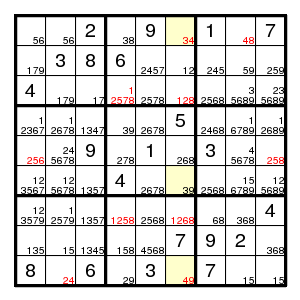`56aA`
`56Aa`
2
`38cC`
9
`34Cc`
1
`48Cc`
7
`179def`
3 8 6
`2457 C E`
`12Dd`
`245 c `
`59gG`
`259`
4
`179  F`
`17hH`
`12578   i `
`2578`
`128`
`2568`
`35689J    `
`235689 j    `
`12367`
`12678`
`1347  c `
`39Cc`
`2678`
5
`2468 C  `
`16789`
`12689`
`256`
`245678 C    `
9
`278 I `
1
`268 l `
3
`45678c    `
`258`
`123567`
`125678`
`1357`
4
`2678`
`39cC`
`2568`
`156789`
`125689`
`123579     F`
`12579    f`
`1357`
`1258`
`2568`
`1268  L `
`68mM`
`368j  `
4
`135`
`15nN`
`1345  C `
`158`
`4568c o `
7 9 2
`368JO `
8
`24Cc`
6
`29cC`
3
`49Cc`
7
`15qQ`
`15Qq`

```d -> F and f -> D
d -> H -> E -> c -> O -> j and J -> o -> C -> e -> h -> D
i -> e -> F and f -> E -> I
f -> g -> q and Q -> G -> F
L -> O -> M and m -> o -> l
A -> n and N -> a
```

We have (4,3)3 > C,c, impossible. So (4,3)!3. Now there are only two possible 3's in row 4, and we can label (4,1)3 with c.

We have (4,3)1 > C,H > h,H, impossible. So (4,3)!1. Now (4,3) has become bivalued, and we can label (4,3)7 with C.

We have (3,9)9 > f,J > f,F, impossible. So (3,9)!9. Now there are only two possible 9's in row 3, and we can label (3,8)9 with f.

We have (7,1)3 > f,J > f,F, impossible. So (7,1)!3. Now there are only two possible 3's in row 7, and we can label (7,3)3 with J.

We have (4,1)7 > C,E > e,E, impossible. So (4,1)!7.

We have (4,7)8 > c,m > M,m, impossible. So (4,7)!8.

We have (7,4)8 > c,m > M,m, impossible. So (7,4)!8.

That were seven more eliminations, but that was all, and the present approach does not help any further. The current state, as also found by Lummox JR is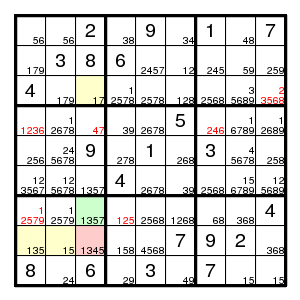## WXYZ-wing

However, Jeff reports that there still is a wxyz-wing. And indeed, each of the four possibilities of the green cell eliminate the possibility 1 for the red cell. Hence (8,3)!1.

That is all progress reported in the thread referred to. What next?

## Almost locked sets

Study the same neighbourhood where this wxyz-wing worked. On the cells (8,1) and (8,2) and one of (7,3),(8,3),(8,4) we have an almost locked set for the three digits 1,3,5. So, let us give names to the three cases (7,3)7, (8,3)4, (8,4)8. When one of these is false there is a strong conclusion.

Label (7,3)7 with r, and (8,4)8 with t, so that r > c,h,j and t > c. Then R = (7,3)!7 > (8,1;8,2;7,3)135 > (8,3)4 = C, so that r = c and R = C. But now both c > h and C > h, which means that h and D hold and H and d are false. Also T = (8,4)!8 > (8,124)135 > (8,9)!3 = j, but also t > c > j, which means that j holds and J is false.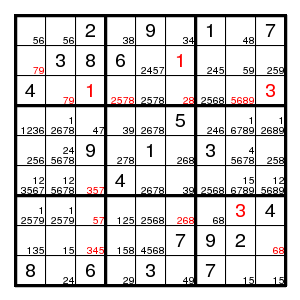## More colouring

Let us redraw the grid, adding a colour s/S for (8,1)3 and (8,3)3, and a colour u/U for (7,4)1 and (8,4)1, and a colour v/V for (3,6). We see that M=O.

`56aA`
`56Aa`
2
`38cC`
9
`34Cc`
1
`48Cc`
7
`79ef`
3 8 6
`2457 C E`
1
`245 c `
`59gG`
`259`
4
`79EF`
1
`2578  i `
`2578`
`28vV`
`2568`
`5689   f`
3
`1236  c `
`12678`
`47cC`
`39Cc`
`2678`
5
`246 C `
`16789`
`12689`
`256`
`245678 C    `
9
`278 I `
1
`268 l `
3
`45678c    `
`258`
`123567`
`125678`
`357s  `
4
`2678`
`39cC`
`2568`
`156789`
`125689`
`12579    F`
`12579    f`
`57Cc`
`125u  `
`2568`
`268 L `
`68mM`
3 4
`135 s `
`15nN`
`345SC `
`158U t`
`4568c m `
7 9 2
`68Mm`
8
`24Cc`
6
`29cC`
3
`49Cc`
7
`15qQ`
`15Qq`

Now C > s because of (8,3) and s > C because of row 6. Hence C = s and S = c. It follows that (6,1)!3 and (8,3)!5. Now there are two possible 5's in column 3, and we can label (6,3)5 with c. Clearly (6,3)!7. Implications:

```E -> c -> O and o -> C -> e
i -> e -> F and f -> E -> I
f -> g -> q and Q -> G -> F
L -> M and m -> l
A -> n -> u and U -> N -> a
C -> n and N -> c
t -> c = S and s = C -> T
t -> u and U -> T
V -> c and C -> v
```

We have (7,4)2 > U,C > N,n, impossible, so (7,4)!2. Now label (7,4)5 with U.

We have (5,2)5 > c,C, impossible, so (5,2)!5.

We have (3,4)2 > I,V,C > V,v, impossible, so (3,4)!2.

We have (7,6)2 > l,V,C > V,v, impossible, so (7,6)!2. Now (7,6) and (7,7) form a double pair for 6,8, so L=M and l=m.

Now (7,5)!68. Introduce a new colour w/W for (7,5). Then w > C.

We have (2,5)5 > w,c,e > C,c, impossible. So (2,5)!5.

Now digit 5 occurs in box 2 in row 3, so (3,7)!5. Introduce a new colour x/X for (3,4)5 and (3,5)5. Introduce a new colour y/Y for (2,7)5 and (6,7)5.

`56aA`
`56Aa`
2
`38cC`
9
`34Cc`
1
`48Cc`
7
`79ef`
3 8 6
`247 CE`
1
`245 cy`
`59gG`
`259`
4
`79EF`
1
`578xi `
`2578 X  `
`28vV`
`268`
`689  f`
3
`1236  c `
`12678`
`47cC`
`39Cc`
`2678`
5
`246 C `
`16789`
`12689`
`256`
`24678 C   `
9
`278CI `
1
`268 m `
3
`45678c    `
`258`
`12567`
`125678`
`35Cc`
4
`2678`
`39cC`
`2568 Y  `
`156789`
`125689`
`12579    F`
`12579    f`
`57Cc`
`15uU`
`25wW`
`68Mm`
`68mM`
3 4
`135 C `
`15nN`
`34cC`
`158U t`
`4568c m `
7 9 2
`68Mm`
8
`24Cc`
6
`29cC`
3
`49Cc`
7
`15qQ`
`15Qq`

```E -> c -> O and o -> C -> e
i -> e -> F and f -> E -> I
f -> g -> q and Q -> G -> F
A -> n -> u and U -> N -> a
C -> n,w and N,W -> c
t -> c,u,M and C,U,m -> T
V -> c -> W -> x -> I,u and i,U -> X -> w -> C -> v
```

Now y > C and Y > C. But that means that C holds and c is false. And from C we also get e,F,n,u. That solves everything. We find

`56`
`56`
2 8 9 3 1 4 7
7 3 8 6 4 1
`25`
`59`
`259`
4 9 1 7 5 2
`68`
`68`
3
`12`
`68`
7 3
`68`
5 4
`19`
`129`
`56`
4 9 2 1
`68`
3 7
`58`
`12`
`58`
3 4 7 9
`25`
`68`
`12568`
9 7 5 1 2
`68`
`68`
3 4
3 1 4 5
`68`
7 9 2
`68`
8 2 6 9 3 4 7
`15`
`15`

The puzzle is completed by simple colouring for the digit 8 (or a Turbot Fish for 8: (5,9)-(5,6)=(4,5)-(8,5)=(8,9)-(5,9)). We see that (5,9)!8, and the rest is singles.